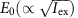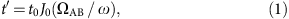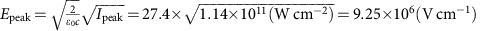## Introduction

Ultrafast control of conduction and magnetic properties in strongly correlated systems1,2,3 has been extensively studied from the perspective of photoinduced insulator-to-metal transitions, or, equivalently, the ‘melting’ of electronic orders (red arrow in Fig. 1a) in Mott insulators4,5,6,7,8,9,10, charge-ordered (CO) systems11,12,13,14 and charge/spin density wave materials6,15,16,17,18,19,20. Recently, the excitation of coherent phonons has been established as a leading strategy for the melting/constructing of electronic orders8,9,15,16,17,18,19,20. On the other hand, the development of strong electric fields (>MV cm−1) of few-cycle optical pulses and recent theoretical studies using dynamical mean-field theory suggest that extreme non-equilibrium electronic states, such as Floquet states, negative temperatures and superconducting states21,22, can be achieved.Figure 1: Melting and freezing of charge motion in α-(ET)2I3 and AC-field-induced charge localization.

In highly non-equilibrium phenomena, reducing the intersite transfer integral t by modulating the site energy under strong continuous-wave (CW)21,23,24,25,26 and pulsed22,26 alternating current (AC) fields E(ω), shown in Fig. 1b, plays an important role. Such an intense modulation of the electronic structure driven by a strong electric field, referred to as ‘dynamical localization’, provides a new strategy for controlling charge motion in strongly correlated materials, which have a competing energy balance between the on-site or intersite Coulomb repulsion and t (ref. 27).

Our target material is a layered organic conductor, α-(ET)2I3 (ET: bis[ethylenedithio]-tetrathiafulvalene), which exhibits a thermal (equilibrium) metal-to-CO insulator transition at TCO=135 K (refs 28, 29, 30, 31, 32) and a photoinduced (non-equilibrium) transition14 as illustrated in Fig. 1a. Efficient photoinduced insulator-to-metal transitions of >200 ET molecules/photon have previously been demonstrated14. However, the optical response of the metallic phase at T>TCO remains unclear.

In this study, we perform pump-probe transient reflectivity measurements for the metallic phase of α-(ET)2I3 using 7 fs 1.5 cycle infrared pulses. Our results demonstrate the optical freezing of charges or, equivalently, photoinduced charge localization in the metallic phase (blue arrow in Fig. 1a). We discuss the mechanism on the basis of theories that have been proposed for non-equilibrium states generated by high-frequency CW and pulsed AC fields.

## Results

### Thermal metal-to-CO insulator transitions

The optical conductivity in the mid- and near-infrared region in Fig. 2a has a clear opening of the CO gap at ~0.1–0.2 eV and a spectral weight transfer to a higher energy at the thermal metal-to-insulator transition29,30. Figure 2b shows the steady-state reflectivity (R) at 140 K (metal: solid red curve) and 40 K (CO: dashed blue curve). As shown in Fig. 2d, at TCO, R abruptly decreases for 0.09 eV (open circles) and increases for 0.64 eV (closed circles) as the temperature decreases. Such a large change (80% at 0.64 eV, 60% at 0.09 eV) in R at the metal-to-insulator transition directly corresponds to the opening of the CO gap and the transfer of the spectral weight to a higher energy of the optical conductivity29,30, as shown in Fig. 2a. Figure 2c shows the spectral differences at different temperatures. The solid curve shows [R(40 K)−R(140 K)]/R(140 K), reflecting the metal-to-insulator change across TCO. The dashed and dashed–dotted curves show [R(190 K)−R(140 K)]/R(140 K) and [R(170 K)−R(140 K)]/R(140 K), exhibiting increases in the electron-lattice temperature up to 190 and 170 K, respectively. Thus, the marked increase of R at >0.55 eV (blue shading) clearly characterizes the metal-to-insulator change across TCO, whereas the rise of the electron-lattice temperature is detected as a reflectivity decrease below 0.65 eV.

### Photoinduced charge localization

Figure 3a shows the transient reflectivity (ΔR/R) spectra for time delays (td) of 30 fs (closed blue circles for an excitation intensity Iex=0.8 mJ cm−2, open blue circles for Iex=0.12 mJ cm−2) and 300 fs (closed black circles for Iex=0.8 mJ cm−2) after excitation by a 7-fs pulse covering the spectral range 0.6–0.95 eV. The spectrum shown by crosses represents ΔR/R at td=300 fs after a 100-fs pulse excitation for Iex=0.8 mJ cm−2. A large increase of RR/R=0.28 at 0.66 eV) was observed at td=30 fs for Iex=0.8 mJ cm−2, as shown by the blue shading in Fig. 3a. The spectral shape of ΔR/R is analogous to that of the temperature differential spectrum [R(40 K)−R(140 K)]/R(140 K) in Fig. 2c, suggesting that a photoinduced metal-to-insulator change occurred. In contrast, as shown by the crosses, the black closed circles and the red shading, the spectral shapes and the magnitude of ΔR/R measured at td=300 fs are analogous to the differential spectrum [R(190 K)−R(140 K)]/R(140 K), reflecting an increase in the electron-lattice temperatures up to 190 K. In such a time domain (300 fs), the lattice system cannot be thermalized and a quasi-thermalized state is formed as a result of the interaction between the charge and various short-period vibrational and optical phonon modes. Therefore, the lattice temperature shown in the reflectivity spectrum at td=300 fs can be defined as a local temperature consisting solely of short-period modes. For Iex=0.12 mJ cm−2, the ΔR/R spectrum shown by the open blue circles indicates that the metal-to-charge-localized change does not occur under weak excitation conditions.

### Charge-ordering gap oscillation along the time axis

The temporal change of the ΔR/R spectrum is plotted as a two-dimensional (probe energy—delay time) map in Fig. 3b. Positive and negative ΔR/R are shown by the blue and red shadings, respectively. The spectrum for td<50 fs, reflecting the transient CO state, oscillates with a period of 20 fs as indicated by the red dotted lines. Then, the spectral shape of ΔR/R markedly changes with the melting of the transient CO and the increase of electron/lattice temperatures shown as the red area in the time scale of 50–100 fs. Such spectral change occurs simultaneously as the oscillation decays. The time profile of ΔR/R sliced at 0.64 eV is shown in Fig. 4a. A positive ΔR/R (solid curve with blue shading) at Iex=0.8 mJ cm−2 persists for td=50 fs after the excitation pulse. Then, ΔR/R becomes negative (red shading), indicating that the photoinduced CO state collapsed because of the increase of electron-lattice temperature. In contrast, for Iex=0.12 mJ cm−2, a positive signal was not detected at 0.64 eV, as shown by the dashed–dotted curve. It is worth noting that the time profile was modulated by the oscillating component with a period of 20 fs. This oscillation, shown in Fig. 4b, can be attributed to the intermolecular charge oscillation reflecting the CO gap, because the time-resolved spectrum of the oscillating component at td=0–40 fs obtained by the wavelet (WL) analysis (blue curve, inset of Fig. 4b) corresponds to the optical conductivity spectrum near the CO gap of ~0.1 eV at 10 K (black curve in the inset).Figure 4: Time profiles of transient reflectivity measured at 0.64 eV.

Although the C=C vibration energy is near the CO gap29,30, the vibrational contribution can be detected separately after td >50 fs, that is, the wavelet spectra at td=80–120 fs indicated by the red curve in the inset of Fig. 4b shows a dip at ~0.15 eV (which equals the vibration peak of the optical conductivity at 140 K (refs 29, 30) in the inset) reflecting the destructive interference between charge motion and vibration33. Therefore, the intense 20 fs oscillation detected before the appearance of the charge-vibration interference dip is attributed to the oscillation of the CO gap. Such CO gap oscillation has been detected in the precursor step to the CO melting33, although the amplitude was much smaller (<1% of the ΔR/R signal) than the present case. Because of the ultrafast decay within 50 fs (corresponding to the energy scale of ħ/50 fs=0.08 eV) of the photoinduced state, we cannot verify the insulating gap below 0.1 eV. However, the gap-like spectral shape at 0.1–0.2 eV, as described above, clearly indicates that the charge motion on the corresponding energy scale is frozen as if the charge distribution on the ET molecules were analogous to that in the CO insulator. In this limited sense, the CO gap is regarded as opened.

Considering that the charge-vibration interference dip appears after the photoinduced charge localization occurs, the vibration-induced mechanism is ruled out, that is, the charge localization is driven by the electronic interaction, although vibration may play some role in stabilizing the transient CO state. Here the lifetime of the transient CO state (<50 fs) is much shorter than that of the coherent phonon-induced state (~1 ps or longer), which has been reported as the melting of a Mott insulator8,9 or the ordering of the transient spin density wave19,20. Such a short lifetime indicates that the transient CO state is not adequately stabilized by vibrational and lattice motions.

From ΔR/R=0.28 at 0.64 eV and the temperature difference [R(40 K)−R(140 K)]/R(140 K)=0.8 at the same energy, the volume fraction of the photoinduced CO state is evaluated to be ~35%. As Iex=0.8 mJ cm−2 corresponds to ~0.0145 photons/ET molecule, the efficiency of this process is 25 ET/photon. This is much lower than that for photoinduced melting of the CO state(>200 ET/photon14), demonstrating that the mechanism of the photoinduced charge localization is completely different from that of the insulator-to-metal transition.

## Discussion

Figure 5a shows ΔR/R at 0.64 eV as a function of the instantaneous electric fieldfor various temperatures. An increase in R (positive ΔR/R) is detectable only for Iex>0.31 mJ cm−2 (E0=5.8 MV cm−1) at 138 K, whereas the negative ΔR/R for Iex<0.31 mJ cm−2 is due to the rise in the electron-lattice temperature. As shown in the inset, the efficiency of the photoinduced charge localization becomes larger near TCO. We can evaluate the positive component of ΔR/R by subtracting the negative component of the time profile shown in Fig. 4a, assuming that the negative component grows exponentially along the time axis (the dashed red curve in Fig. 4a). The resultant positive component exhibits a nonlinear increase as a function of E0, as shown in Fig. 5b.

In non-equilibrium states induced by a strong AC field E(ω), t is reduced for CW21,23,24,25 and pulsed22,26 light. According to the dynamical localization theory23,24,25,26 for a CW AC field, the effective t (= t′) can be represented as,where t0 is the transfer integral for zero field. Here, ΩABe rAB·E0/ħ, where E0 and ω, respectively, denote the amplitude and angular frequency of the electric field of the light; E(t)=E0 sin(ωt); rAB is the vector from the A site to its nearest-neighbour B site of α-(ET)2I3, as shown in Fig. 1a; and e is the elementary charge. Equation (1) is satisfied for CW light. In addition, on the basis of the numerical solution of the time-dependent Schrödinger equation for the two-site tight-binding model and a model for a CO molecular conductor26, this relation has recently been shown to be satisfied by pulsed light, with respect to the efficiency of the intersite electronic transition (that is, the energy increment due to the pulsed light). Also, in the dynamical mean-field theory calculations, the change in the electronic state into one with modified t appears within the time scale of a few optical cycles after the sudden application of a CW AC field21. This fact also suggests that the dynamical localization functions transiently with the few-cycle pulse. If we use the parameter rABcosθ=5.4 angstroms (refs 34, 35); where θ represents the relative angle between rAB and E, t is reduced, as it is proportional to the zeroth order Bessel function J0, and becomes zero at 40 MV cm−1AB/ω=2.40), as shown in Fig. 5c. From Fig. 5c, we can estimate that the change in J0t′ is approximately 10% for a typical instantaneous field of 9.3 MV cm−1 (Iex=0.8 mJ cm−2).

To demonstrate the instability of the metallic phase induced by this 10% change in t, we roughly estimated the change of TCO by changing t. Figure 6a shows the temperature dependence of the hole densities (ρH) at the A and A′ molecules in Fig. 1a as a function of the normalized temperature T/TCO, calculated using the Hartree–Fock approximation for an extended Hubbard model36. A charge disproportionation occurs below T/TCO=1, as indicated by the closed (A: charge rich) and open (A′: charge poor) circles for the original t0. We also calculated the ρH versus T/TCO relation with changing t, where the rectangles, upward triangles and downward triangles show the calculated values for 0.95t0, 0.9t0 and 0.85t0, respectively. Figure 6b illustrates that the change in TCO (=ΔTCO/TCO) is proportional to the decrease in t (=−Δt /t), indicating that a 10% change in t causes a 12% change in TCO from 135 to 152 K. Thus, TCO increases across the measured temperature (138 K).

For our experimental conditions (<12 MV cm−1), 1/t′ (in units of 1/t0) shows a nonlinear dependence on E0 (red curve in Fig. 5b). This nonlinear dependence of 1/t′ on E0 is consistent with the relation between ΔR/R and E0 (closed circles in Fig. 5b). Considering that R reflects the metal-to-insulator transition (Fig. 2b), it is reasonable to assume that ΔR/R is proportional to the efficiency of the transient charge localization. As such, the E0 dependence of ΔR/R, can be described by 1/t′.

According to recent studies on sub-cycle asymmetric pulses22, the momentum shift of the band structure is given by the ‘dynamical phase’ φ=∫ dtE(t) (ref. 22). However, in the present case, this is estimated to be φ=1.66 × 10−4[rad] at most, which is much smaller than φ=π/2[rad], and is too small to cause a detectable momentum shift. Such shift would be detected if the width of the asymmetric pulse were shorter than ~3 fs at this wavelength. Another problem lies in the mechanism driving the reduced t, which should be applicable to events before the excitation ends, that is, ~7 fs. The reason why the photoinduced CO persists for ~50 fs after the 7 fs pulse is unclear at the moment. Further experiments and theoretical considerations will be needed to clarify this issue.

In summary, this report demonstrates a large reflectivity increase (>25%) and a coherent CO gap oscillation along the time axis indicating the opening of a CO gap by the 9.3 MV cm−1 electric field of a 1.5 cycle, 7 fs near-infrared pulse in an organic conductor α-(ET)2I3. The plausible mechanism for such a dramatic change in the electronic state is the reduction of t (~10%) driven by this strong high-frequency field.

## Methods

### Sample preparation

Single crystals of α-(ET)2I3 (2 × 1 × 0.1 mm) were prepared using a method described in a previous study28.

### 7-fs Infrared pulse generation

A broadband infrared spectrum for the 7-fs pulse covering 1.2–2.3 μm, shown by the orange curve in Fig. 2a, was obtained by focusing a carrier-envelope phase stabilized idler pulse (1.7 μm) from an optical parametric amplifier (Quantronix HE-TOPAS pumped by Spectra-Physics Spitfire-Ace) onto a hollow fibre set within a Kr-filled chamber (Femtolasers). Pulse compression was performed using both active mirror (OKO Technologies, 19-ch linear MMDM) and chirped mirror (Femtolasers, Sigma-Koki) techniques. The pulse width derived from the autocorrelation of the generated second harmonic was 7 fs, which corresponds to 1.5 optical cycles. The instantaneous electric field on the sample surface (excitation diameter 200 μm) for a typical excitation intensity can be evaluated as, where Ipeak=1.14 × 1011 W cm−2 represents the peak power for an excitation intensity Iex of 0.8 mJ cm−2.

### Transient reflectivity measurements

We performed transient reflectivity experiments using both 7 and 100 fs pulses. The excitation photon energies for 7 and 100 fs pulses were 0.6–0.95 eV (7 fs) and 0.89 eV (100 fs), respectively. In the transient reflectivity measurement using a 7-fs pulse, the probe pulse reflected from the sample was detected by InGaAs detector (New-Focus model 2034) after passing through a spectrometer (Bunkoukeiki, M10). The pump-on and pump-off were alternately switched by the feed-back-controlled optical chopper (New Focus, Model 3501), synchronized with the laser driver. Each probe shot was sampled using boxcar integrators (Stanford Research, SR250). After normalization by a reference pulse, the observed intensity of respective shots was recorded in the PC.